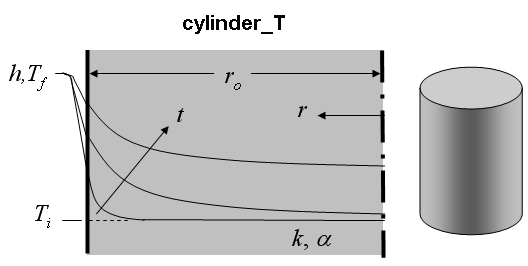Contents - Index

Cylinder_TFunction cylinder_T(r, time, T_i, T_f, alpha, k, h, r_o) returns the temperature  ([C], [K], [F], or [R]) within a long right circular cylinder with the exterior walls (r=r_o) exposed to convection.

Inputs:
r - radial position [m] or [ft]
time - time relative to beginning of convection [sec]
T_i - initial temperature of cylinder [C], [K], [F], or [R]
T_f - bulk fluid temperature [C], [K], [F], or [R]
alpha - thermal diffusivity [m^2/s] or [ft^2/hr]
k - thermal conductivity of cylinder [W/m-K] or [Btu/hr-ft-R]
h - heat transfer coefficient [W/m^2-K] or [Btu/hr-ft^2-R]
r_o - outer radius of cylinder [m] or [ft]

Notes:
Function cylinder_T determines the dimensionless length, and non-dimensional Biot and Fourier numbers. It then calls the function cylinder_T_ND which determines theta as described in Section 3.5.2 of Nellis and Klein.  Theta is then resolved by cylinder into the temperature.

Example:
\$UnitSystem SI K Pa J
r=0.02 [m]
time=3000 [sec]
T_i=303 [K]
T_f=363 [K]
alpha=8.608e-5 [m^2/s]
k= 233 [W/m-K]
h= 45 [W/m^2-K]
r_o= 0.1 [m]
T=cylinder_T(r, time, T_i, T_f, alpha, k, h, r_o)

{Solution: T=340.7 [K]}

Transient Conduction Index